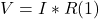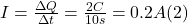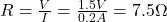## The voltage across a resistor is found to be 1.5 V. It is also found that there is a charge of 2 Coulombs passing through the resistor withi

Question

The voltage across a resistor is found to be 1.5 V. It is also found that there is a charge of 2 Coulombs passing through the resistor within 10 seconds. What is the value of the resistor in ohms

in progress 0
1 month 2021-09-05T16:11:16+00:00 1 Answers 0 views 0

R = 7.5 Ω

Explanation:

• If the resistor is in the linear zone of operation, the resistance must obey Ohm’s Law:• By definition, the current flowing through the resistor, is equal to the charge passing through the resistor, per unit time.
• So, we can write the following expression for the current I:• From (1) and (2) we can solve for R, as follows:• The value of the resistor is 7.5 Ω.